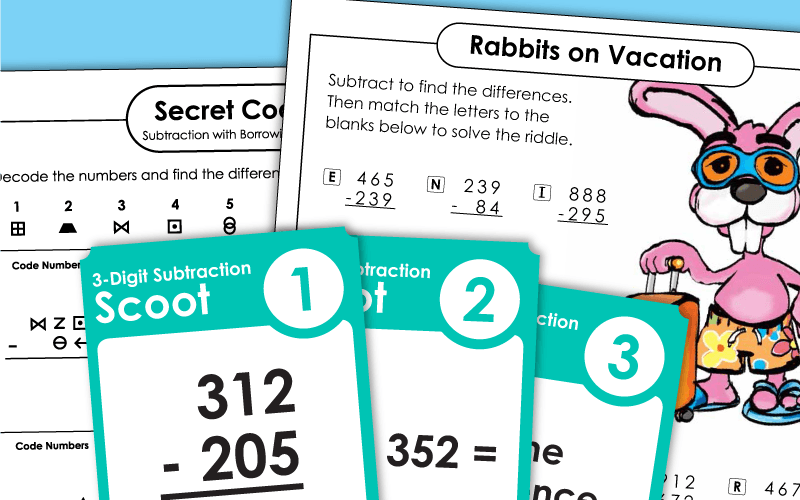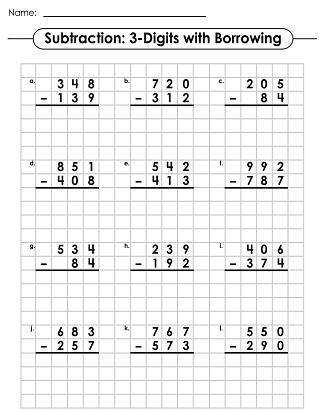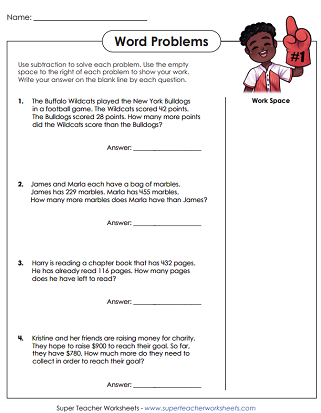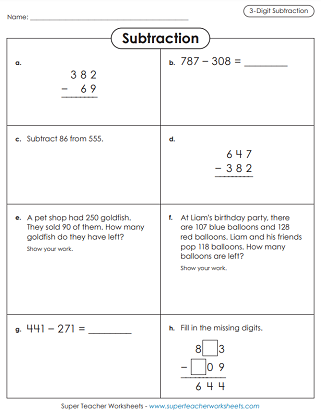# Subtraction: 3-Digit Numbers

This page has printables for teaching three-digit subtraction. There are lots of traditional worksheets, as well as card games and math riddle puzzles.## 3-Digit SubtractionWorksheets

Practice subtracting 3-digit numbers with borrowing / regrouping.
Riddle: How does a rabbit travel? To find the answer, solve three-digit subtraction problems. Then match the answers with the letters to decode the solution to the riddle.
Subtract pairs of three-digit numbers to find the differences. Most problems require borrowing across zero. Then match up the letters to the answers to find the answer to a funny riddle.
Solve a variety of subtraction problems, including word problems, vertical and horizontal equations, and missing digit questions.
These subtraction story problems involve borrowing. Students work with 2 and 3-digit numbers on this activity.
On the top of this page, there are pictures of shapes with 3-digit numbers in them. Students find differences between pairs of shapes. For example: Subtract the number in the pentagon from the number
in the octagon.
Solve the subtraction problems and color the answers on the bingo boards.  Can you get a bingo?
Color the picture of the knight, dragon, and castle. Then solve the subtraction problems.
Use base 10 blocks (place value blocks) to solve the subtraction problems on this worksheet.
These completed subtraction problems have mistakes in them. Figure out what's wrong with each one and explain.
Here's a fun activity that kids enjoy. First, students decode the picture symbols using the cipher key near the top of the page. Then they subtract the numbers they've decoded.
This worksheet is similar to the one above, except all problems require students to subtract across zero. (example: 700-382)
First solve 16 3-digit subtraction problems. Then cut out the numbered puzzle pieces. Then attach each numbered piece onto the correct problem. This reveals a mystery picture of a school bus with students.
Cut out the math cards. Solve the subtraction problems. Then sort the answers into "even differences" and "odd differences." After that, students glue the math cards in the correct columns on the t-chart.
Subtract to find the missing addends  (example: 71 + __ = 143)
Practice 3-digit Subtracting with borrowing / regrouping.  Check by adding.
These subtraction problems have 3-digit quantities and require borrowing. The worksheet features pictures of sea animals.
Subtract the three-digit numbers to find the differences. All problems require some borrowing. There are also two word problems.
Rewrite each problem vertically, then solve.  Students required to "line up the digits" correctly.
Solve the 2-digit numbers from the 3-digit numbers.  Then attach the puzzle pieces in the correct positions.  Picture shows two kids sledding down a hill.
Practice 3-digit Subtracting problems that require borrowing across zero

## Graph Paper Math

This subtraction worksheet has pictures of cute dragons.  3-digits with borrowing.
Graph paper subtraction will ensure kids can line up their place value columns perfectly.
Subtracting on graph paper gives kids plenty of room to write their numbers and illustrates place value

Here's a complete class set of thirty task cards with 3-digit subtraction problems on them. Use them to create your own classroom learning center. You can also use them to play math games, such as Scoot! Or place them under your document camera during your math lesson.
In this game, students move to each desk in the classroom and solve 3-digit subtraction problems.
In this game, students must figure out the correct locations for the missing digits in each subtraction problem. Includes 12 task cars and a student answer page.

Complete the bar models (sometimes known as part-part-whole illustrations or tape diagrams) by adding or subtracting.
These tape diagrams (bar models) have space on the right-hand side for students to show their work. This is a 2-page file.
How do bees get to school? In order to decode the answer, student answer a series of 3-digit addition and subtraction problem. (Answer: On the school buzz!)
Students must determine whether they need to add or subtract to solve each problem.
Students must determine whether they need to add or subtract to solve each problem.

## Mental Subtraction(Subtraction Patterns)

Why was the music teacher locked out of her classroom? To find the answer, solve the mental-math subtraction problems. Each problem has 2, 3, 4, or 5-digit numbers. (example: 900-200)

## Subtraction Worksheet Generator

Subtraction Worksheet Generator

Make custom worksheets with your own 2, 3, 4, or 5-digit subtraction problems. Choose vertical or horizontal problems. You can even write your own title and choose a custom header.

4-Digit Subtraction

More Subtraction

Printable subtraction worksheets with single and multi-digit problems. This index page will direct you to 2-digit problems, subtraction without borrowing, or even decimal subtraction.

Math Worksheets

Download and print thousands of math worksheets on a variety of topics, including addition, multiplication, symmetry, bar graphs, counting money, telling time, and patterns.

## Sample Worksheet ImagesMy Account
Site Information